# Tamilnadu Board Class 10 English Grammar Sentence Pattern

## Tamilnadu State Board Class 10 English Grammar Sentence Pattern

There are five components in a sentence.

1. Subject;
2. Verb;
3. Object;
4. Complement;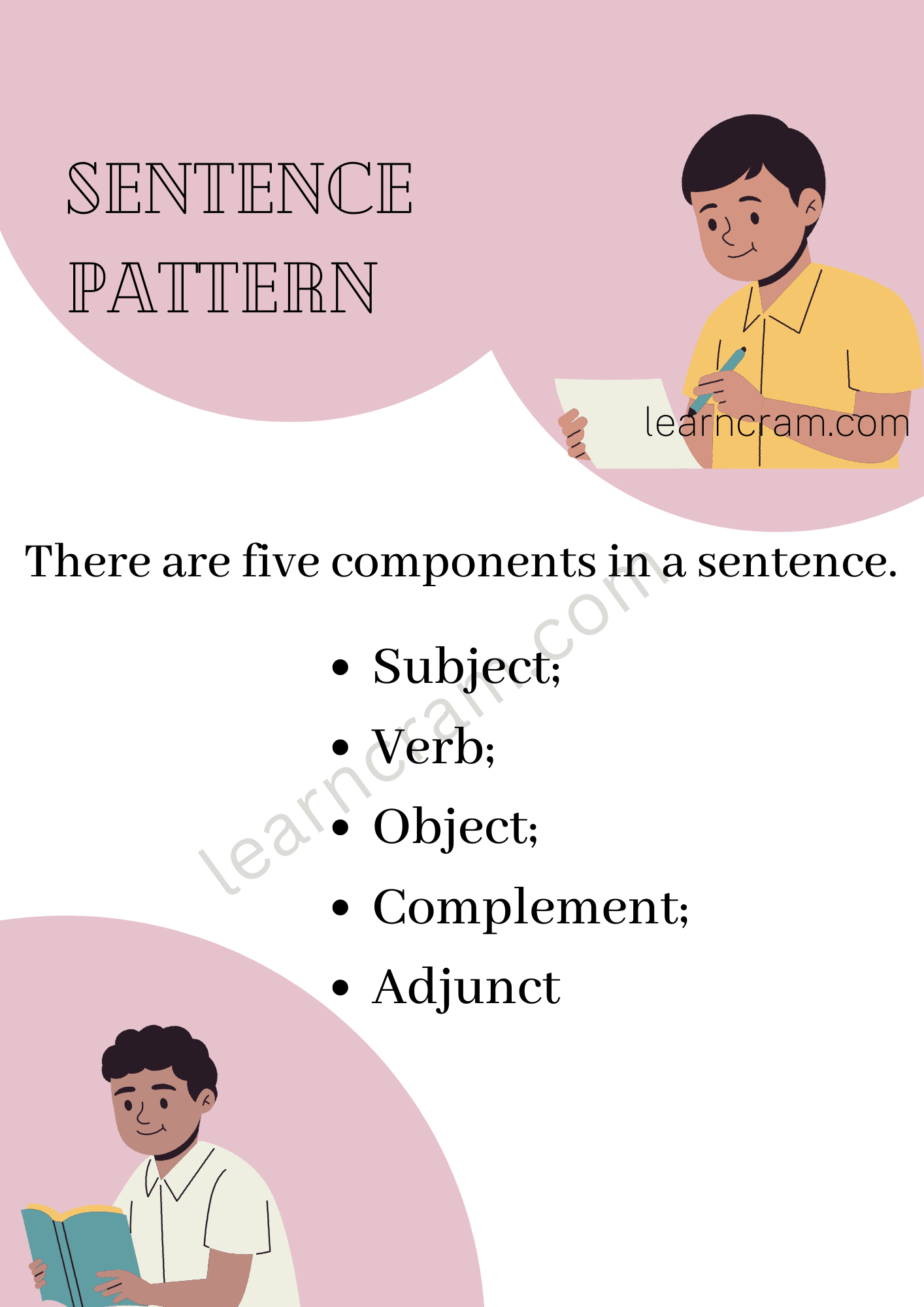1. Subject:
Subject – S – consists of nouns or pronouns – occurs before a verb.2. Verb:
Verb-
V – consists of
(a) auxiliaries
(b) finite verbs.

(a) Auxiliaries:

1. am, is are, was, were
3. does, do, did
4. Modals: can, could; will, would; shall, should; may, might; must
5. Semi-Modals / Quasi Modals: dare to; need to; used to; ought to

(b) Finite verbs – denote action:
e.g.,
sing, play, write, make, dance, leave, create, etc.
verbs occur after the subject (or) before the object.

Examples of S + V pattern: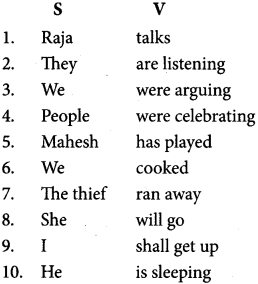3. Object:
Object – O – consists of nouns or noun phrases or noun clauses.
(a) DO – Direct Object
– answers the question ‘what’ after V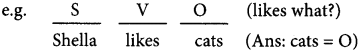(b) IO – Indirect ObjectExamples of S V O pattern: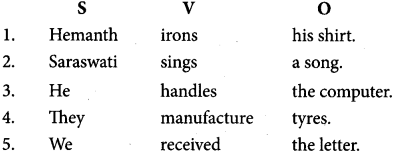Examples of S V O DO pattern:Examples of S V O IO pattern: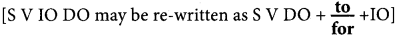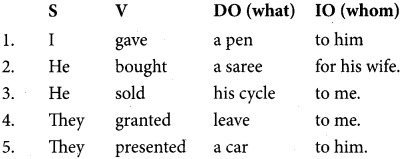4. Complement:
Complement – C – from the word ‘complete’
– complete the meaning in the sentence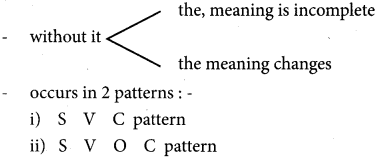i) In S V C pattern, the complement C
– complements the subjects
– without C, the sentence is incomplete or the sentence changes its meaning.
– “to be” forms, grow, become, seems for a verb.– the same person/thing
– without C, incomplete meaning.

But in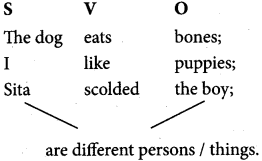ii) in S V O C pattern:
– the complement tells about the object
– the complement and object are of the same person or thing.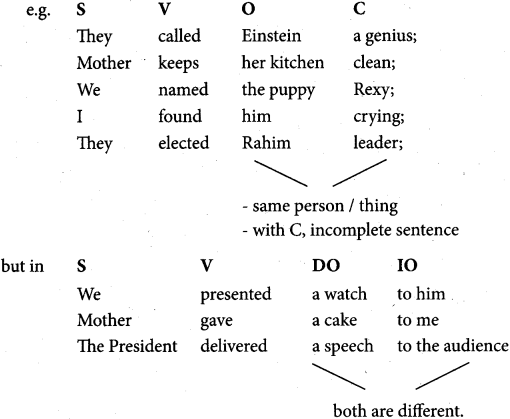Adjunct – A – answers the questions where? when ? how ? why?
– Absence of ‘A’ does not change the meaning of the sentence.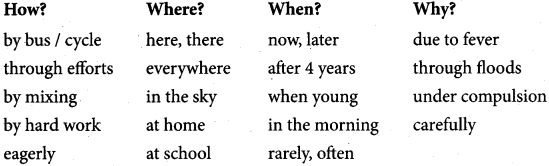1. She / comes I every day. – S V A
2. Sit / here. – V A
3. He / drives / the car / slowly. – S V O A
4. Muthu / has been I an officer / for 10 years. – S V C A
5. We / present I gifts / to our friends / on birthdays. – S V D O IO A
6. This morning / at five / I I heard / a noise. – A A S V O
7. We / rarely I go / to the beach I during weekends. – S A V A A
8. Every year I on New Year’s eve / they / distribute / cake and sweets I to all the guests. – A A S V DO IO
9. Every morning / at five I the Mullah/chants/ prayers / at the mosque I without fail. – A A S V O A A
1o. In the end,) the judge I declared I the accused / innocent, after the trial. – A S V O C A

Exercises
Identify the pattern of the following sentence:

Sentence Pattern Question 1.
He was going to school.
(a) S V O
(b) S V A
(c) S V C A
(b) S V A

Question 2.
I met my friend at the market.
(a) S V I O D O
(b) S V O C
(c) S V O A
(c) S V O A

Question 3.
Velu grew tired ãfter the match.
(a) S V C A
(b) S V I O D O
(c) S V O C
(a) S V C tile n fg[pA

Question 4.
(a) S V O C
(b) S V I O D O
(c) S V O A
(a) S V O C

Question 5.
They named this child Ria.
(a) S V A C
(b) S V O C
(c) S V C A
(b) S V O C

Question 6.
Ram’s father is a lawyer.
(a) S V C
(b) S V A
(c) S V I O D O
(a) S V C

Question 7.
He sang me a song melodiously.
(a) S V O C
(b) S V O A
(c) S V IO DO A
(c) SV IO DO A

Question 8.
This shoe is large.
(a) S V O
(b) S V C
(c) S V A
(b) S V C

Sentence Pattern Exercises for Class 10 Question 9. His father gave him his school bag.
(a) S V C
(b) S V O C
(c) S V IO DO
(c) S V IO DO

Question 10.
The player entered the room.
(a) S V O
(b) S V A
(c) S V C
(b) S V A

Question 11.
Eve – Teasers must be punished severely.
(a) S V A
(b) S V C
(c) S V O
(a) S V A

Question 12.
We wear woolen clothes in winter season.
(a) S V O C
(b) S V IO DO
(c) S V O A
(c) S V O A

Question 13.
I shall meet you tomorrow.
(a) S V O
(b) S V IO DO
(c) S V O A
(c) S V O A

Question 14.
(a) S V O A
(b) S V C A
(c) S V IO DO
(a) S V O A

Question 15.
They named the child Prem.
(a) S V A C
(b) S V O C
(c) S V C A
(c) S V C A

Question 16.
We completed the work on time.
(a) S V O C
(b) S V A C
(c) S V O A
(c) S V O A

Question 17.
The meeting ended with a vote of thanks.
(a) S V A
(b) S V C
(c) S V O